# Using MathScript and Formula Nodes in NI LabVIEW

Publish Date: Feb 11, 2020 | 20 Ratings | 3.75 out of 5 | Print | 2 Customer Reviews | Submit your review

## Overview

The Formula Node in the LabVIEW software is a convenient, text-based node you can use to perform complicated mathematical operations on a block diagram using the C++ syntax structure. It is most useful for equations that have many variables or are otherwise complicated. The text-based code simplifies the block diagram and increases its readability. Furthermore, you can copy and paste existing code directly into the Formula Node rather than recreating it graphically.

In addition to text-based equation expressions, the Formula Node can accept text-based versions of if statements, while loops, for loops, and do loops, which are familiar to C programmers. These programming elements are similar but not identical to those you find in C programming.

The MathScript Node implements similar functions but with the additional functionality of a full .m file compiler, making it useful as a textual language for signal processing, analysis, and math. LabVIEW MathScript is generally compatible with .m file script syntax, which is widely used by alternative technical computing software. For LabVIEW 2009 and later, the LabVIEW MathScript features are released separately in the LabVIEW MathScript RT Module.

### Using the Formula Node

Complete the following steps to create a VI that computes different formulas depending on whether the product of the inputs is positive or negative.

1. Selecting File»New VI to open a blank VI.
2. Place a Formula Node on the block diagram.
1. Right-click on the diagram and navigate to Programming»Structures»Formula Node.
2. Click and drag the cursor to place the Formula Node on the block diagram.
3. Right-click the border of the Formula Node and select Add Input from the shortcut menu.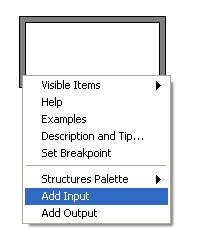1. Label the input variable x.
2. Repeat steps 3 and 4 to add another input and label it y.
3. Right-click the border of the Formula Node and select Add Output from the shortcut menu.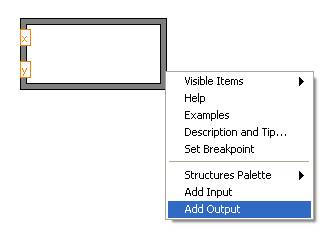1. Create two outputs and name them z1 and z2, respectively.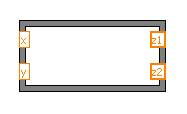Note: It is considered good programming practice to keep the inputs on the left border and the outputs on the right border of the Formula Node. This helps you follow the data flow in your VI and keep your code organized.

1. Enter the expressions below in the Formula Node. Make sure that you complete each command with a semicolon. Notice, however, that the if statement does not require a semicolon after the first line.

if (x*y>0)
z1 = 3*x**2 - 2*y**3;
else z1 = 0;
z2 = sinh(z1);

1. Create controls and indicators for the inputs and outputs.
1. Right-click on each input and select Create»Control from the shortcut menu.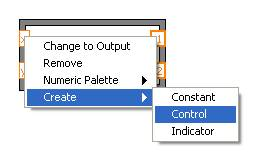1. Right-click on each output and select Create»Indicator from the shortcut menu.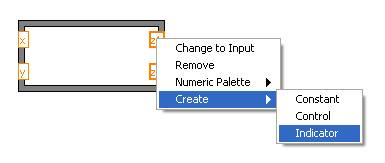1. Place a While Loop with a stop button around the Formula Node and the controls. Be sure to include a Wait (ms) function inside the loop to conserve memory usage. Your block diagram should appear as follows.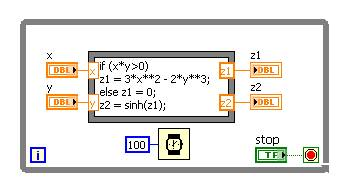1. Click the Run button to run the VI. Change the values of the input controls to see how the outputs change.

In this case, the Formula Node helps minimize the space required on the block diagram. Accomplishing the same task without the use of a Formula Node requires the following code.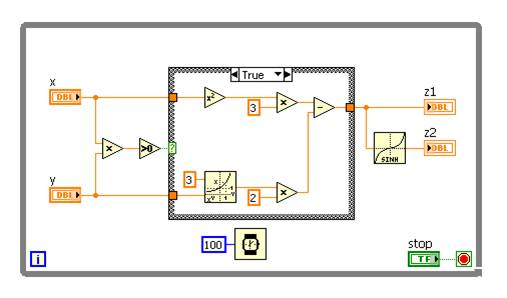### Resources

For more information on the Formula Node syntax or the functions available, see the LabVIEW Help by pressing the <Ctrl-H> keys while you are developing your code. This opens the Context Help window, which includes information about the feature that your mouse is hovering over. In the Context Help window, select Detailed help for more information.

### Using the MathScript Node

Complete the following steps to create a VI that performs various operations on a 1D array in LabVIEW.

1. Open a blank VI from the toolbar by selecting File»New VI.
2. Place a MathScript Node on the block diagram.
1. Right-click on the diagram and navigate to Programming»Structures»MathScript Node.
2. Click and drag the cursor to place the MathScript Node on the block diagram.
3. In the same manner as you implemented in the Formula Node exercise, right-click on the border and select Add Input from the shortcut menu. Label the input x.
4. Right-click the border and select Add Output from the shortcut menu. Repeat this process to create three outputs labeled y, y1, and d. For LabVIEW 2010 and later select Add Output»Undetected Variable.
5. Place an array of numeric controls on the front panel. Label the array x and wire it to the x input of the MathScript Node on the block diagram.
6. In the MathScript Node, enter the following expressions:

y = x.^2;
y1 = y(1);
d = dot(x,y);

1. Create indicators for each of the three outputs by right-clicking each output and selecting Create»Indicator from the shortcut menu.
2. Place a While Loop with a stop button around the MathScript Node and the controls. Be sure to include a Wait (ms) function inside the loop to conserve memory usage. Your block diagram should appear as follows.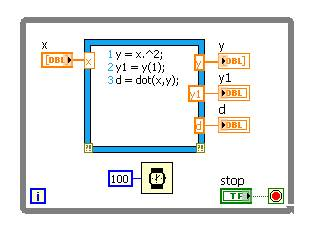1. On the front panel, expand the arrays to show multiple elements. With the cursor, grab the bottom middle selector of the array and drag it down to show multiple elements.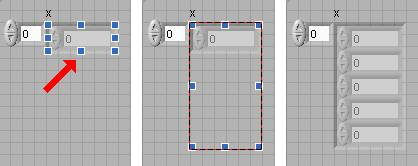1. Begin by placing a 1, 2, and 3 in the first three elements of the x control. Your front panel should look similar to the one below. Note that the fourth and fifth elements are grayed out. This is because they are not initialized. You can initialize them by clicking inside the cell and entering a value. To uninitialize a cell, right-click the element and select Data Operations»Delete Element from the shortcut menu.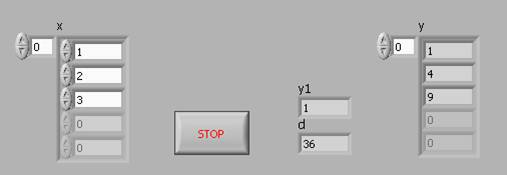1. Click the Run button. Change the values of the elements in the array to see how the outputs change.

### Resources

For more information on the Formula Node syntax or the functions available, see the LabVIEW Help.  Remember you can access the help by using the Context Help window (Ctrl-H). View the related links below to learn more about the MathScript Node.

Customer Reviews
2 Reviews | Submit your review

Using MATLAB  - Oct 16, 2012

When using MATLAB script (see comment above) you must right click on x input and y output and select Choose Data Type, 1-D Array of Real. You must also create an array of numeric indicators for y. You must also have a licensed copy of MATLAB on your computer.

Warning! Name Change  - Jun 21, 2012

This tutorial is old, so it uses the name "MathScript." I can't find the node called "MathScript" in the current version of LabVIEW. I think the same function is now called "MATLAB Script," which is also accurate, since it looks like MATLAB code. I found it under Mathematics>>Scripts and Formulas>>Script Nodes>>MATLAB script.

### Ratings

Rate this document